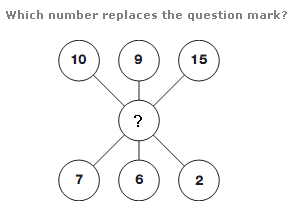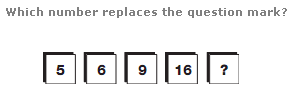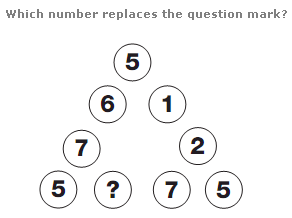# Puzzles - Number puzzles

### Exercise :: Number puzzlesAnswer : 2 Explanation : Split the diagram into 3 vertical columns. The sum of the numbers in each column equal 17.Answer : 31 Explanation : As you move to the right, double the previous number and subtract 4, then 3, then 2, then 1 to give the next number along.Answer : 7 Explanation : Start anywhere on the diagram, and move clockwise, calculating the sum of every third circle. This always equals 15.